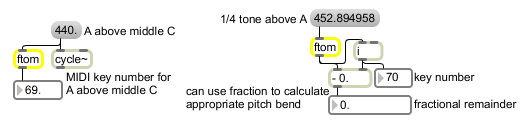ftom

Convert frequency to a MIDI note number

Description

ftom converts frequency, given in Hertz, to a MIDI pitch or note number (0-127).

Arguments

Name Type Opt Description
output-format float opt If a float value is present, the ftom object outputs floating-point values. By default, it outputs int values.

Messages

 int frequency [int] A frequency value. The corresponding MIDI pitch value (from 0 to 127) is sent out the outlet. float frequency [float] A frequency value. The corresponding MIDI pitch value (from 0 to 127) is sent out the outlet. list list of frequencies [list] A list of frequency values. Corresponding MIDI pitch values (from 0 to 127) for each list item are sent out the outlet.

Attributes

Name Type g/s Description
base float Sets the "base frequency" used when converting frequency values (e.g., A = 440.). The default is 440.

Output

float: The MIDI note value that corresponds to the input frequency. When an input frequency falls between two equal tempered pitches, the value is rounded to the nearest int when ftom is used in its default int mode. When ftom is used in the optional float mode, the fractional part of the float is included. The fractional part could be used to calculate an additional pitch offset for applying MIDI pitch bend.
int: The MIDI note value that corresponds to the input frequency. When an input frequency falls between two equal tempered pitches, the value is rounded to the nearest int when ftom is used in its default int mode. When ftom is used in the optional float mode, the fractional part of the float is included. The fractional part could be used to calculate an additional pitch offset for applying MIDI pitch bend.

ExamplesFind the MIDI key number to play the same pitch as an MSP oscillator